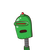# Shilpa deposited same amount of money in two different banks, which pay simple interest at the rate of 10% and 12%, respectively.

Shilpa deposited same amount of money in two different banks, which pay simple interest at the rate of 10% and 12%, respectively. After 3 yr total interest received by her is Rs. 3300. The total amount deposited is a) Rs. 10000

c) Rs. 12000 e) None of these

b) Rs. 11000 d) Rs. 12250​

### 1 thought on “Shilpa deposited same amount of money in two different banks, which pay simple interest at the rate of 10% and 12%, respectively.”

1.